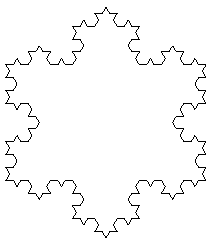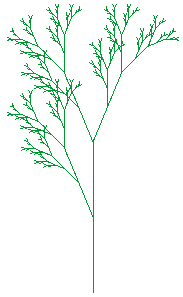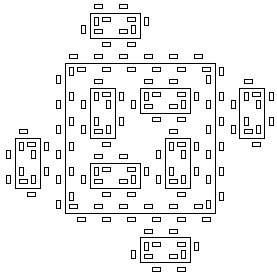# L-Systems

L-Systems are described in the following book:

• Przemyslaw Prusinkiewicz and Aristid Lindenmayer
The Algorithmic Beauty of Plants
Springer-Verlag, New York, 1990

Available software:

• lsystem.c   -- source for Unix/X11 or MS-DOS/VGA
• lsystem.exe -- executable for MS-DOS/VGA
• demos.zip   -- dozens of example L-System definitions
Given a correct L-System definition, the program can:
1. Display an L-System on X11 (Unix) or on a VGA screen (MS-DOS)
2. Produce a PostScript figure, by specifying -p as a command line argument

## ExamplesKoch snowflake

```seed    F + + F + + F          # Initial sequence of symbols
rule    F -> F - F + + F - F   # Symbol rewrite rule
delta   60                     # Angle of rotation in degrees
theta   60                     # Initial angel in degrees
leng    400                    # Length of line element
iter    3                      # Number of iterations
ratio   3                      # Scaling of length/iteration
x       0                      # Initial horizontal position
y       -235                   # Initial vertical position
```Shrub

```ignore  X                      # X will be ignored
seed    X
rule    F -> F F
rule    X -> F [+ X] F [+ X] - X
delta   22.5
theta   90
leng    230
iter    6
ratio   2
x       0
y       -230
red     0.0                    # color component between 0 and 1
green   0.6                    #
blue    0.2                    #
```Islands

```move    f                      # f produces invisible line
seed    F + F + F + F
rule    F -> F+f-FF+F+FF+Ff+FF-f+FF-F-FF-Ff-FFF
rule    f -> f f f f f f
delta   90
theta   90
leng    236
iter    2
ratio   6
x       128
y       -128
```

Any letter may be used as a non-terminal. For any used letter, there has to be a a corresponding rule. The program is case sensitive for letters used in rules and seed.

Use of color is optional.

Use of ratio is optional (default = 1). The value of leng is divided by ratioiter. For many (but not all) figures, ratio can be defined such that, when the value for iter is altered, no other values need to be adjusted.

## Not (yet?) implemented

• Parameters could be used to specify length, width, color, etc.:
```F(x) -> F(x * 1.41)
```
• Parametric conditionals, such as:
```F(x <  .5) -> F(x * 1.41)
F(x >= .5) -> [ + F(x)] - F(x)
```
• Probabilistic conditionals, such as:
```F(.8, .2) -> F
-> [ - F ] [ + F ] F
```